# 一文帶你瞭解 Python 中的繼承知識點

theme: v-green highlight: a11y-dark

# 1 類繼承

Python 是面向物件的程式語言，因此支援面向物件的三大特性之一：繼承。

dir(object) ['class', 'delattr', 'dir', 'doc', 'eq', 'format', 'ge', 'getattribute', 'gt', 'hash', 'init', 'init_subclass', 'le', 'lt', 'ne', 'new', 'reduce', 'reduce_ex', 'repr', 'setattr', 'sizeof', 'str', 'subclasshook']

```

Python 中繼承類的語法如下：

```python class SubClass(SuperClass):```

## 1.1 繼承的簡單例子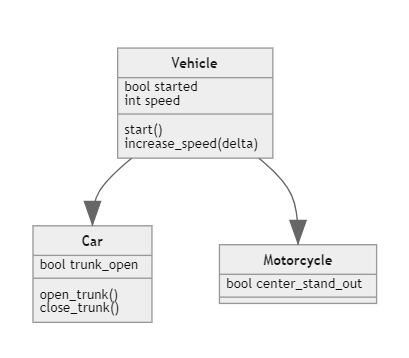Python 程式碼實現如上繼承關係，如下：

```python class Vehicle:

``````def __init__(self, started =  False, speed = 0):
self.started = started
self.speed = speed

def start(self):
self.started = True
print("啟動，皮皮蝦我們走")

def stop(self):
self.speed = 0

def increaseSpeed(self, delta):
if self.started:
self.speed += delta
print("Vroooooom!")
else:
print("還沒啟動呢！")
``````

class Car(Vehicle): trunk_open = False def open_trunk(self): self.trunk_open = True def close_trunk(self): self.trunk_open = False ```

```python class Motorcycle(Vehicle): def __init__(self, center_start_out = False): self.center_start_out = center_start_out super().__init__() def start(self): print("Sorry, out of fuel!")```

```python class Vehicle:

``````def __init__(self, started =  False, speed = 0):
self.started = started
self.speed = speed

def start(self):
self.started = True
print("啟動，皮皮蝦我們走")

def stop(self):
self.speed = 0

def increaseSpeed(self, delta):
if self.started:
self.speed += delta
print("Vroooooom!")
else:
print("還沒啟動呢！")
``````

class Car(Vehicle): trunk_open = False def open_trunk(self): self.trunk_open = True def close_trunk(self): self.trunk_open = False

class Motorcycle(Vehicle): def init(self, center_start_out = False): self.center_start_out = center_start_out super().init()

V = Vehicle() C = Car() M = Motorcycle()

print(type(V)) print(type(C)) print(type(M))

print("摩托車是交通工具的例項嗎？",isinstance(M, Vehicle)) ```

`super()` 函式返回一個代表父類的臨時物件。這用於訪問父類的方法和屬性。在 `super()` 函式的幫助下，我們還可以訪問被覆蓋的方法。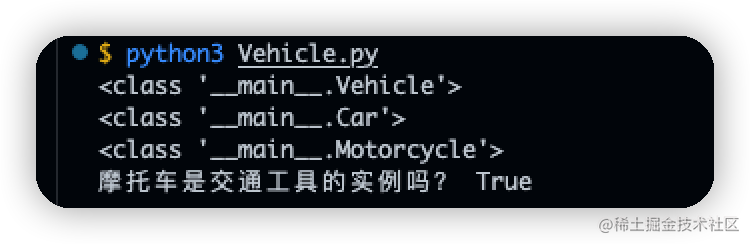# 2 繼承的型別

## 2.1 單繼承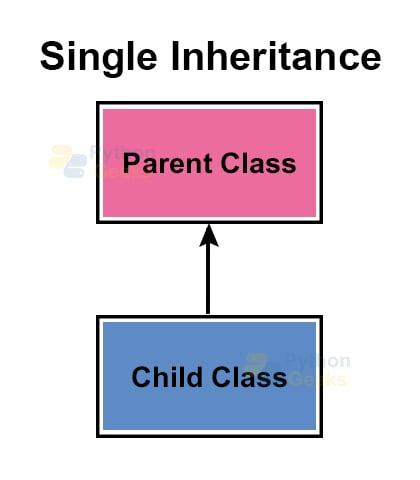```python class Animal: print("我是動物")

class Cat(Animal): print("我是貓") ```

## 2.2 多繼承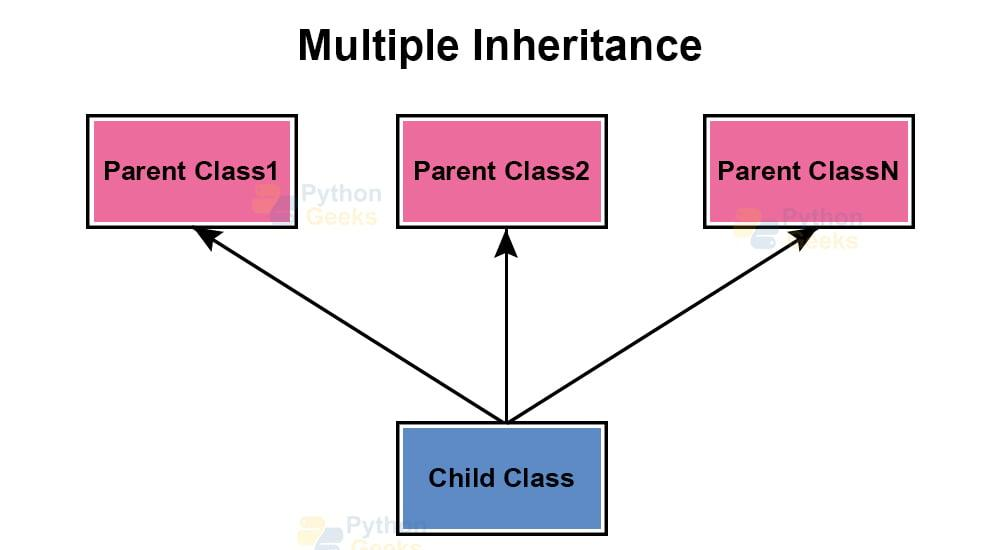```python class A: def init(self, name): self.name = name

def introduction(self): print("我叫", self.name)

class B: def init(self, age): self.age = age

def introduction(self): print("我今年 %d 歲了" % self.age)

class C(A, B): pass

c = C(18) c.introduction()

```

`我叫 18`

## 2.3 多級繼承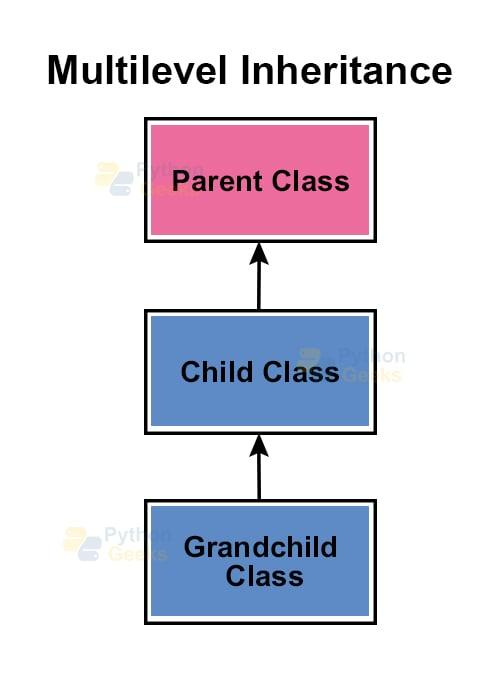```python class Parent: str1 = "Python"

class Child(Parent): str2 = " is the best programming?"

class GrandChild(Child):

``````def get_str(self):
print(self.str1 + self.str2)
``````

person = GrandChild() person.get_str()

```

`Python is the best programming?`

## 2.4 分層繼承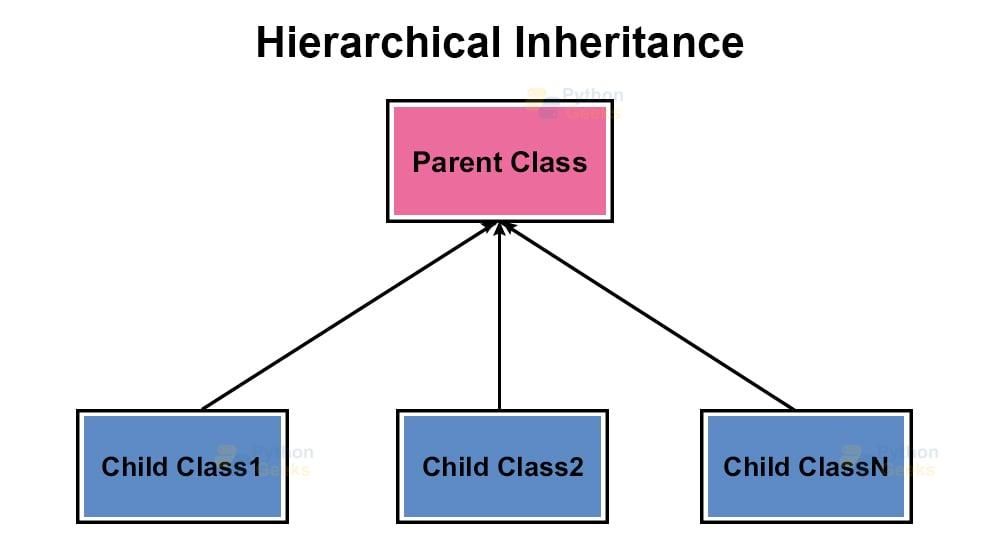```python class SuperClass: x = 2022

class SubClass1(SuperClass): pass

class SubClass2(SuperClass): pass

class SubClass3(SuperClass): pass

a = SubClass1() b = SubClass2() c = SubClass3() print(a.x, b.x, c.x)

```

`(2022, 2022, 2022)`

## 2.5 Python中的混合繼承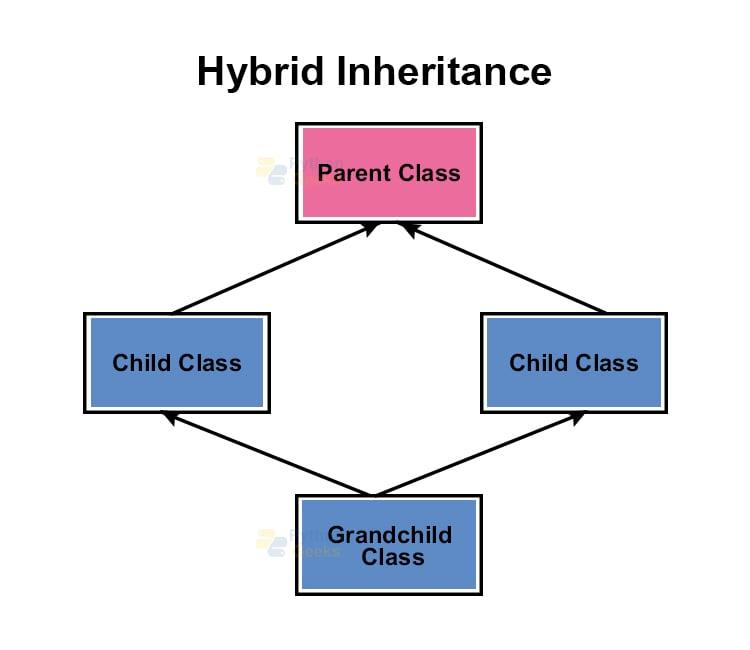```python class X: num = 2023

class A(X): pass

class B(A): pass

class C(A): pass

class D(B, C): pass

ob = D() print(D.num)

```

`2023`

「其他文章」